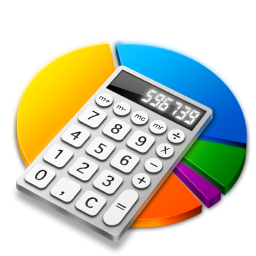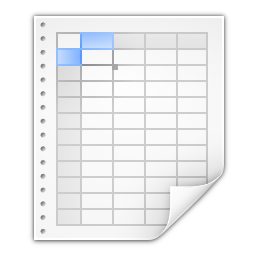SSuite Office Software

Providers of Safe and Trusted Software with Pure Visual Simplicity

Working with spreadsheet data using SSuite Office applications

Here is a quick update to spreadsheet header formatting: { Row and Column Names }

To change, edit, or add a name to any cell's row or column header, just double click on the cell's row or column header e.g. A - B - C etc.

A dialog window will appear, just enter a name and click on the "OK" button. The header's name will now change.

Just remember that this feature is not supported in MS Excel. They have as yet not added it to their very expensive software apps. So take notice of this fact if you are going to save your spreadsheets in the Excel format - *.xls.Merging data in an Accel Spreadsheet

Simple Concatenation of Data

The following formula combines data from cells A1, B1 and C1.

Write this formula into another column, such as column D1:

=A1&B1&C1

The following formula combines data from cells A1, B1 and C1 and adds spaces between each set of data. Write this formula into another column, such as column D1:

=A1&" "&B1&" "&C1

---------------------------------------------------------------------

How to compare data in two columns to find duplicates in Accel

To use a worksheet formula to compare the data in two columns, follow these steps:

1. Start Accel.2. In a new worksheet, enter the following data (leave column B empty):

A1: 1 B1: C1: 3

A2: 2 B2: C2: 5

A3: 3 B3: C3: 8

A4: 4 B4: C4: 2

A5: 5 B5: C5: 0

3. Type the following formula in cell B1:

=IF(ISERROR(MATCH(A1,\$C\$1:\$C\$5,0)),"",A1)

4. Now drag the selection to cells B1:B5.The duplicate numbers are displayed in column B, as in the following example:

A1: 1  B1:     C1: 3

A2: 2  B2: 2  C2: 5

A3: 3  B3: 3  C3: 8

A4: 4  B4:     C4: 2

A5: 5  B5: 5  C5: 0

---------------------------------------------------------------------

How to find data in an Accel table

This article uses a sample worksheet to illustrate Accel's built-in functions, for example referencing a name from column A and returning the age of that person from column C. To create this worksheet, enter the following data into a blank Accel worksheet.

You will type the value that you want to find into cell E2. You can type the formula in any blank cell in the same worksheet.A         B           C       D         E

1     Name     Dept  Age      Find Value

2     Henry     501     28       Mary

3     Stan       201     19

4     Mary       101     22

5     Larry      301     29

Terms, Definitions, and Examples:

Table_Array - The whole lookup table - A2:C5

Lookup_Value - The value to be found in the first column of Table_Array - E2

Lookup_Array

-or-

Lookup_Vector - The range of cells that contains possible lookup values - A2:A5

Col_Index_Num - The column number in Table_Array the matching value should be returned for - 3 (third column in Table_Array)

Result_Array

-or-

Result_Vector - A range that contains only one row or column. It must be the same size as Lookup_Array or Lookup_Vector - C2:C5

Range_Lookup - A logical value (TRUE or FALSE). If TRUE or omitted, an approximate match is returned. If FALSE, it will look for an exact match - FALSE

Top_Cell - This is the reference from which you want to base the offset. Top_Cell must refer to a cell or range of adjacent cells. Otherwise, OFFSET returns the #VALUE! error value.

Offset_Col - This is the number of columns, to the left or right, that you want the upper-left cell of the result to refer to. For example, "5" as the Offset_Col argument specifies that the upper-left cell in the reference is five columns to the right of reference. Offset_Col can be positive (which means to the right of the starting reference) or negative (which means to the left of the starting reference).

---------------------------------------------------------------------

Functions used in Accel:

LOOKUP()

The LOOKUP function finds a value in a single row or column and matches it with a value in the same position in a different row or column.

The following is an example of LOOKUP formula syntax:

=LOOKUP(Lookup_Value,Lookup_Vector,Result_Vector)

The following formula finds Mary's age in the sample worksheet:

=LOOKUP(E2,A2:A5,C2:C5)

The formula uses the value "Mary" in cell E2 and finds "Mary" in the lookup vector (column A). The formula then matches the value in the same row in the result vector (column C). Because "Mary" is in row 4, LOOKUP returns the value from row 4 in column C (22).

Note - The LOOKUP function requires that the table be sorted.

---------------------------------------------------------------------

VLOOKUP()

The VLOOKUP or Vertical Lookup function is used when data is listed in columns. This function searches for a value in the left-most column and matches it with data in a specified column in the same row.The following example uses a table with unsorted data.

The following is an example of VLOOKUP formula syntax:

=VLOOKUP(Lookup_Value,Table_Array,Col_Index_Num,Range_Lookup)

The following formula finds Mary's age in the sample worksheet:

=VLOOKUP(E2,A2:C5,3,FALSE)

Note - You can use VLOOKUP to find data in a sorted or unsorted table.

The formula uses the value "Mary" in cell E2 and finds "Mary" in the left-most column (column A). The formula then matches the value in the same row in Column_Index. This example uses "3" as the Column_Index (column C). Because "Mary" is in row 4, VLOOKUP returns the value from row 4 in column C (22).

---------------------------------------------------------------------

INDEX() and MATCH()

You can use the INDEX and MATCH functions together to get the same results as using LOOKUP or VLOOKUP.

The following is an example of the syntax that combines INDEX and MATCH to produce the same results as LOOKUP and VLOOKUP in the previous examples:

=INDEX(Table_Array,MATCH(Lookup_Value,Lookup_Array,0),Col_Index_Num)

The following formula finds Mary's age in the sample worksheet:

=INDEX(A2:C5,MATCH(E2,A2:A5,0),3)

The formula uses the value "Mary" in cell E2and finds "Mary" in column A. It then matches the value in the same row in column C. Because "Mary" is in row 4, the formula returns the value from row 4 in column C (22).

Note - If none of the cells in Lookup_Array match Lookup_Value ("Mary"), this formula will return #N/A.

---------------------------------------------------------------------

OFFSET() and MATCH()

You can use the OFFSET and MATCH functions together to produce the same results as the functions in the previous example.

The following is an example of syntax that combines OFFSET and MATCH to produce the same results as LOOKUP and VLOOKUP:

=OFFSET(top_cell,MATCH(Lookup_Value,Lookup_Array,0),Offset_Col)

This formula finds Mary's age in the sample worksheet:

=OFFSET(A1,MATCH(E2,A2:A5,0),2)

The formula uses the value "Mary" in cell E2 and finds "Mary" in column A. The formula then matches the value in the same row but two columns to the right (column C). Because "Mary" is in column A, the formula returns the value in row 4 in column C (22).